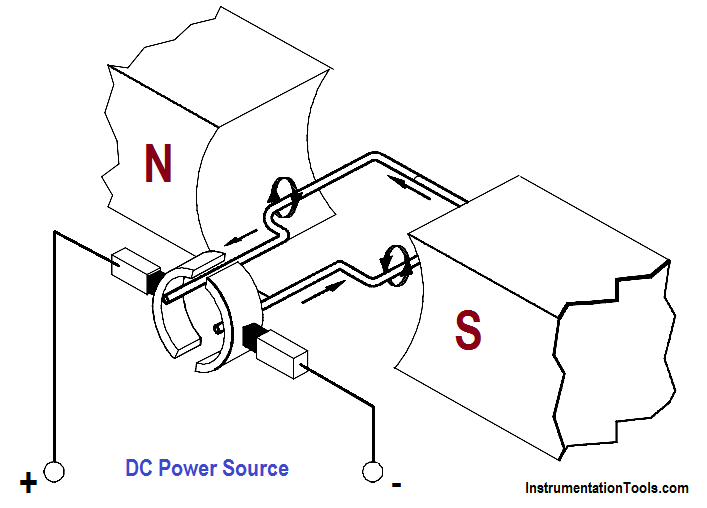# DC Motor Torque

Torque is defined as that force which tends to produce and maintain rotation. The function of torque in a DC motor is to provide the mechanical output or drive the piece of equipment that the DC motor is attached to.

When a voltage is applied to a motor, current will flow through the field winding, establishing a magnetic field. Current will also flow through the armature winding, from the negative brush to the positive brush as shown in Figure 5.Figure 5 : Armature Current in a Basic DC Motor

Since the armature is a current carrying conductor in a magnetic field, the conductor has a force exerted on it, tending to move it at right angles to that field. Using the left-hand rule for current carrying conductors, you will see that the magnetic field on one side is strengthened at the bottom, while it is weakened on the other side.

Using the right-hand rule for motors, we can see that there is a force exerted on the armature which tends to turn the armature in the counter-clockwise direction. The sum of the forces, in pounds, multiplied by the radius of the armature, in feet, is equal to the torque developed by the motor in pound-feet.

It is evident from Figure 5 that if the armature current were reversed, but the field were the same, torque would be developed in the opposite direction. Likewise, if the field polarity were reversed and the armature remained the same, torque would also be developed in the opposite direction.

The force that is developed on a conductor of a motor armature is due to the combined action of the magnetic fields. The force developed is directly proportional to the strength of the main field flux and the strength of the field around the armature conductor. As we know, the field strength around each armature conductor depends on the amount of current flowing through the armature conductor. Therefore, the torque which is developed by the motor can be determined using Equation.

T = K Φ Ia

where

T  = torque, lb-ft
K  = a constant depending on physical size of motor
Φ = field flux, number of lines of force per pole
Ia = armature current